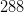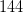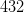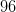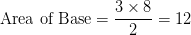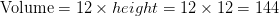# SSAT Upper Level Math : How to find the volume of a polyhedron

## Example Questions

← Previous 1

### Example Question #1 : How To Find The Volume Of A Polyhedron

Find the volume of a square pyramid that has a height ofand a side length of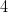.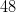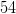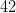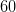Explanation:

The formula to find the volume of a square pyramid is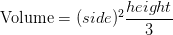So plugging in the information given from the question,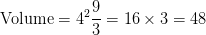### Example Question #2 : How To Find The Volume Of A Polyhedron

Find the volume of a square pyramid with a height of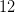and a length of a side of its square base of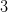.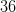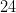Explanation:

The formula to find the volume of a square pyramid isSo plugging in the information given from the question,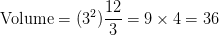### Example Question #3 : How To Find The Volume Of A Polyhedron

Find the volume of a regular hexagonal prism that has a height of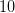. The side length of the hexagon base is.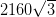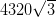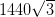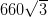Explanation:

The formula to find the volume of a hexagonal prism is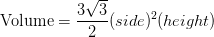Plugging in the values given by the question will give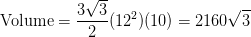### Example Question #4 : How To Find The Volume Of A Polyhedron

In terms of, find the volume of a regular hexagonal prism that has a height of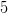. The hexagon base has side lengths of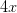.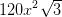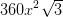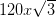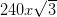Explanation:

The formula to find the volume of a hexagonal prism isPlugging in the values given by the question will give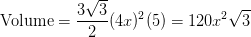### Example Question #5 : How To Find The Volume Of A Polyhedron

Find the volume of a regular tetrahedron with a side length of.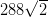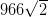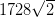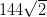Explanation:

The formula to find the volume of a tetrahedron is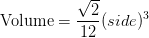Plugging in the information given by the question gives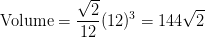### Example Question #6 : How To Find The Volume Of A Polyhedron

Find the volume of a regular octahedron that has a side length of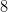.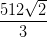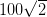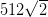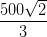Explanation:

Use the following formula to find the volume of a regular octahedron: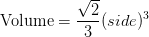Plugging in the information from the question,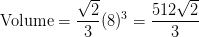### Example Question #7 : How To Find The Volume Of A Polyhedron

Find the volume of a regular octahedron that has a side length of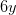.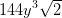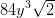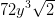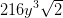Explanation:

Use the following formula to find the volume of a regular octahedron:Plugging in the information from the question,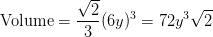### Example Question #8 : How To Find The Volume Of A Polyhedron

Find the volume of a regular hexahedron with a side length of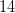.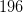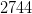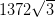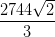Explanation:

A regular hexahedron is another name for a cube.

To find the volume of a cube,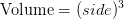Plugging in the information given in the question gives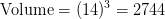### Example Question #9 : How To Find The Volume Of A Polyhedron

Find the volume of a regular octahedron with side lengths of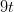.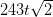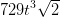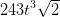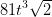Explanation:

Use the following formula to find the volume of a regular octahedron:Plugging in the information from the question,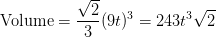### Example Question #10 : How To Find The Volume Of A Polyhedron

Find the volume of a prism that has a right triangle base with leg lengths ofandand a height of.